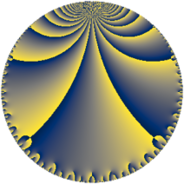# Properties

 Label 124.6.fLevel $124$ Weight $6$ Character orbit 124.f Rep. character $\chi_{124}(33,\cdot)$ Character field $\Q(\zeta_{5})$ Dimension $56$ Newform subspaces $1$ Sturm bound $96$ Trace bound $0$

# Related objects

## Defining parameters

 Level: $$N$$ $$=$$ $$124 = 2^{2} \cdot 31$$ Weight: $$k$$ $$=$$ $$6$$ Character orbit: $$[\chi]$$ $$=$$ 124.f (of order $$5$$ and degree $$4$$) Character conductor: $$\operatorname{cond}(\chi)$$ $$=$$ $$31$$ Character field: $$\Q(\zeta_{5})$$ Newform subspaces: $$1$$ Sturm bound: $$96$$ Trace bound: $$0$$

## Dimensions

The following table gives the dimensions of various subspaces of $$M_{6}(124, [\chi])$$.

Total New Old
Modular forms 332 56 276
Cusp forms 308 56 252
Eisenstein series 24 0 24

## Trace form

 $$56 q + 2 q^{3} - 58 q^{5} + 104 q^{7} - 1234 q^{9} + O(q^{10})$$ $$56 q + 2 q^{3} - 58 q^{5} + 104 q^{7} - 1234 q^{9} - 509 q^{11} - 117 q^{13} + 89 q^{15} - 3504 q^{17} + 262 q^{19} + 352 q^{21} - 2448 q^{23} + 49618 q^{25} + 14324 q^{27} - 9888 q^{29} - 12771 q^{31} + 27699 q^{33} + 13840 q^{35} + 76096 q^{37} + 33520 q^{39} - 4843 q^{41} - 40778 q^{43} + 56692 q^{45} + 38922 q^{47} - 17126 q^{49} - 69292 q^{51} - 41728 q^{53} - 172096 q^{55} + 57066 q^{57} - 58198 q^{59} + 176328 q^{61} - 37444 q^{63} + 143863 q^{65} + 9812 q^{67} - 9250 q^{69} - 67356 q^{71} - 63512 q^{73} - 198012 q^{75} - 74257 q^{77} + 137651 q^{79} + 196077 q^{81} + 156427 q^{83} + 238828 q^{85} - 558144 q^{87} - 99292 q^{89} - 243609 q^{91} - 325925 q^{93} - 75077 q^{95} - 476340 q^{97} + 745812 q^{99} + O(q^{100})$$

## Decomposition of $$S_{6}^{\mathrm{new}}(124, [\chi])$$ into newform subspaces

Label Dim $A$ Field CM Traces $q$-expansion
$a_{2}$ $a_{3}$ $a_{5}$ $a_{7}$
124.6.f.a $56$ $19.888$ None $$0$$ $$2$$ $$-58$$ $$104$$

## Decomposition of $$S_{6}^{\mathrm{old}}(124, [\chi])$$ into lower level spaces

$$S_{6}^{\mathrm{old}}(124, [\chi]) \cong$$ $$S_{6}^{\mathrm{new}}(31, [\chi])$$$$^{\oplus 3}$$$$\oplus$$$$S_{6}^{\mathrm{new}}(62, [\chi])$$$$^{\oplus 2}$$